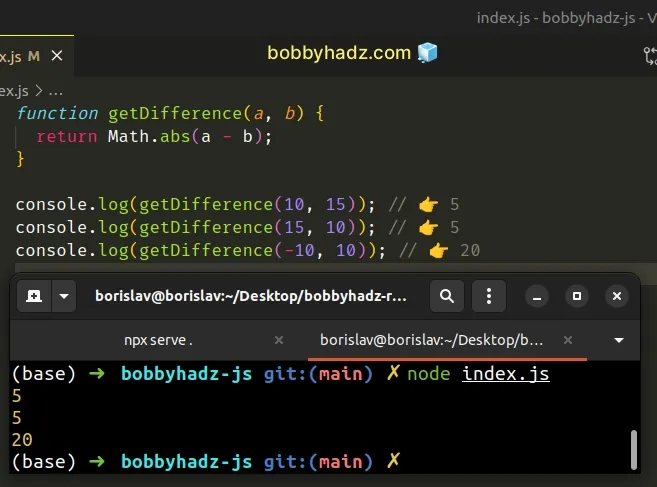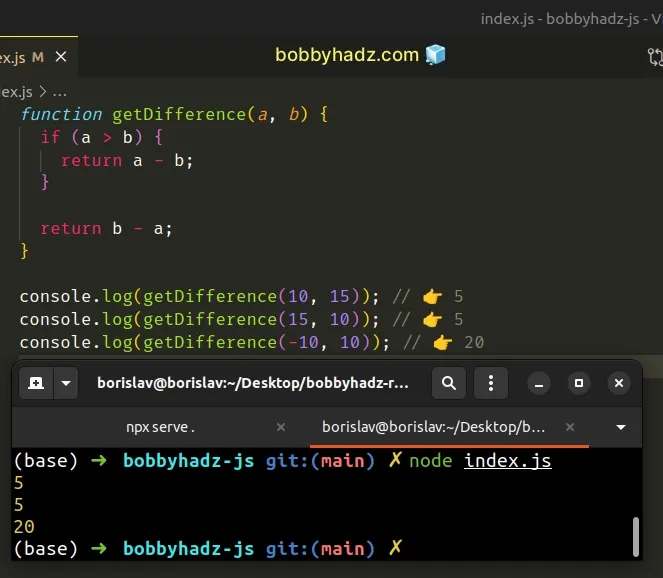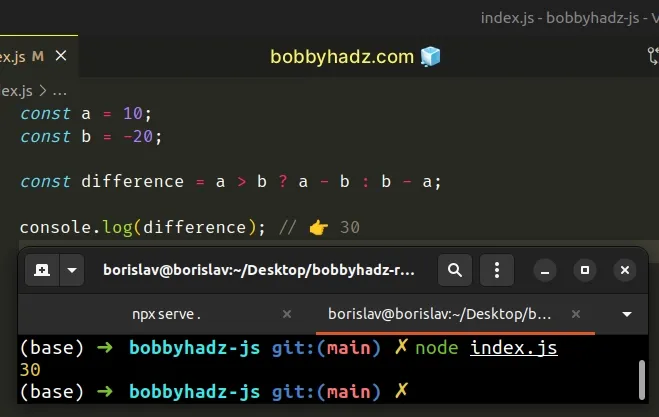# Get the Difference between Two Numbers in JavaScriptLast updated: Jan 6, 2023
2 min## #Get the difference between Two Numbers in JavaScript

To get the difference between two numbers, subtract the first number from the second and pass the result to the `Math.abs()` method.

The `Math.abs()` method returns the absolute value of a number.

index.js
```Copied!```function getDifference(a, b) {
return Math.abs(a - b);
}

console.log(getDifference(10, 15)); // 👉️ 5
console.log(getDifference(15, 10)); // 👉️ 5
console.log(getDifference(-10, 10)); // 👉️ 20
``````The Math.abs() method returns the absolute value of a number.

In other words, it returns the number's distance from zero.

Here are some examples:

index.js
```Copied!```console.log(Math.abs(-3)); // 👉️ 3
console.log(Math.abs(-3.5)); // 👉️ 3.5
console.log(Math.abs(-0)); // 👉️ 0
console.log(Math.abs(3.5)); // 👉️ 3.5
console.log(Math.abs('-3.5')); // 👉️ 3.5
``````

The `Math.abs()` method returns the provided number if it's positive or zero and the negation of the number if it's negative.

The difference between the numbers is always going to be a positive number, so the `Math.abs()` method is perfect for this use case.

An alternative approach is to use an `if` statement.

## #Get the Difference between Two Numbers using an `if` statement

This is a two-step process:

1. Use an `if` statement to check which number is greater.
2. Subtract the lower number from the greater number.
index.js
```Copied!```function getDifference(a, b) {
if (a > b) {
return a - b;
}

return b - a;
}

console.log(getDifference(10, 15)); // 👉️ 5
console.log(getDifference(15, 10)); // 👉️ 5
console.log(getDifference(-10, 10)); // 👉️ 20
``````The `if` statement checks if the first number is greater than the second.

If the condition is met, we subtract the lower number from the greater and return the result.

Otherwise, we know that the numbers are equal or the second number is greater, so we do the inverse.

This is a bit more readable than using the `Math.abs()` method because the method is very rarely used and not many developers are familiar with it.

The code sample could also be shortened by using a ternary operator.

## #Get the difference between two numbers using a ternary operator

This is a two-step process:

1. Use the ternary operator to determine which number is greater.
2. Subtract the lower number from the greater number and return the result.
index.js
```Copied!```const a = 10;
const b = -20;

const difference = a > b ? a - b : b - a;

console.log(difference); // 👉️ 30
``````The ternary operator is very similar to an `if/else` statement.

If the expression to the left of the question mark is truthy, the operator returns the value to the left of the colon, otherwise, the value to the right of the colon is returned.

If you have to do this often, define a reusable function.

index.js
```Copied!```function getDifference(a, b) {
return a > b ? a - b : b - a;
}

console.log(getDifference(10, 15)); // 👉️ 5
console.log(getDifference(15, 10)); // 👉️ 5
console.log(getDifference(-10, 10)); // 👉️ 20
``````

The `getDifference` function takes 2 numbers as parameters and returns the difference between the numbers.